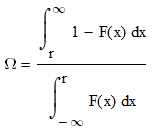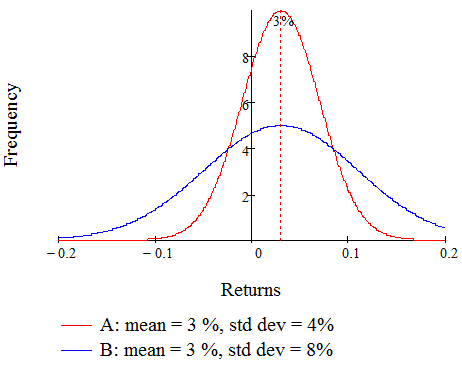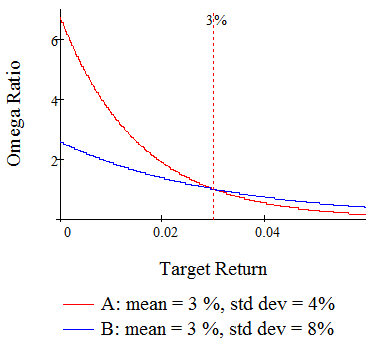The Omega Risk Measure

Traditional investment performance benchmarks quantify how much investors could potentially lose, given the variance (or downside-variance) of the portfolio. These include the Sharpe Ratio and the Sortino Ratio, which generally favor investments with a lower downside risk.

However, most investors have specific investment goals; they’re aiming for a specific return. Also, many non-traditional investments such as hedge funds and options have non-normal distributions – their risk is not well-described by the variance. Moreover, standard deviation tends to weight upside and downside variance equally, in sharp contrast to the risk preference of most investors.

A better way of describing downside risk (at least, for most investors) is the probability-weighted average return below a target return.  There are various names for this, including the Expected Shortfall.

It’s not a big leap to accept that the most appropriate upside gain measure is the probability-weighted average return above a target return.

Taking this once step further, the most appropriate overall risk measure is the probability-weighted average return above a target return divided by the probability-weighted return below a target return. In other words, this is the ratio of the expected gains to the expected losses.

Previously, these ratios have been calculated parametrically, which places an assumption on the shape of the returns distribution. Shadwick and Keating (2001), however, proposed a non-parametric gains-to-losses ratio called the Omega Ratio.

Unlike the Sharpe Ratio, the Omega Ratio does not assume any specific returns distribution. Instead, it captures all the information in the historical returns distribution, and is defined by this equationF(x) is the cumulative distribution of returns, and r is the desired threshold return.

This definition neatly captures skew and kurtosis, and other higher moments present in the returns distribution. This means the Omega Ratio is suitable for assessing the risk of investments whose returns distributions are highly asymmetric or bi-modal. This includes situations described by the Allais Paradox (for example, the risk of buying or selling one lottery ticket).

By utilizing a desired threshold return, the Omega Ratio can be used to model risk for investors with widely differing requirements. For example, a greedy investor may desire a higher target return but will have to accept a higher risk.

Shadwick and Keating (2001) also recommend that investors consider the Omega Ratio for a range of target returns (as opposed to the traditional single estimate for the Sharpe or Sortino Ratio). This is because beyond a certain point, investors have to accept a greater potential downside to achieve a greater potential upside. The Omega Ratio tells you where this point is.

Consider the following returns distributions for two investments, A and B.While both have the same mean return of 3%, investment A has a standard deviation of 4%, while B has a standard deviation of 8%. Based on this information alone, an investor would naturally prefer investment A given its lower volatility.

However, if the investor has a target return of 3%, then investment B would be preferred because it has a high probability of delivering this return or higher.

Now, for the same two funds A and B, let’s plot the Omega Ratio for a range of target returns.For a target return of 3% or less, fund A has the higher Omega Ratio. However, for a target return of more than 3%, fund B is superior. Both curves intersect when the Omega Ratio is equal to 1 (that is, when the target return equals the mean return).

The Sharpe Ratio, on the other hand, will always prefer investment A because of its lower standard deviation.